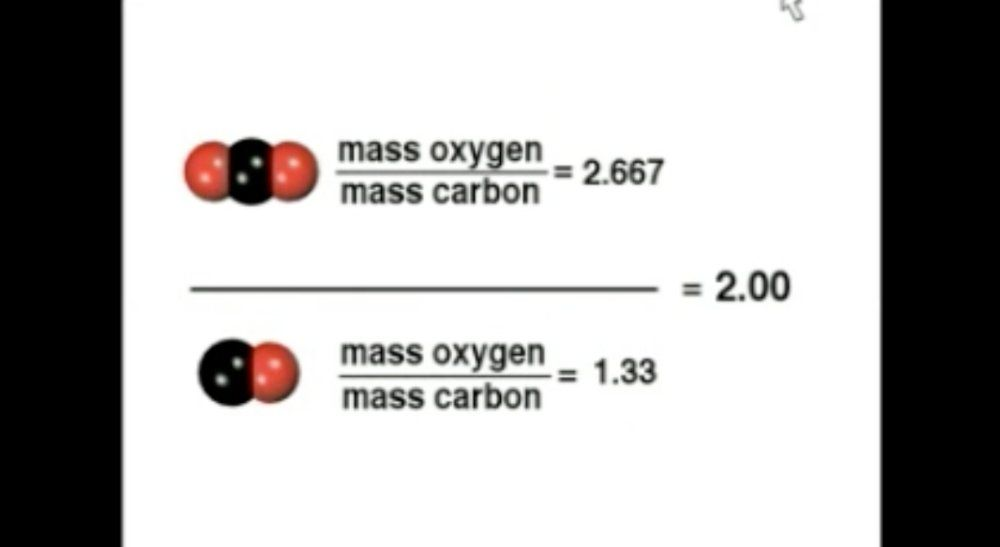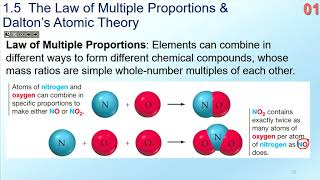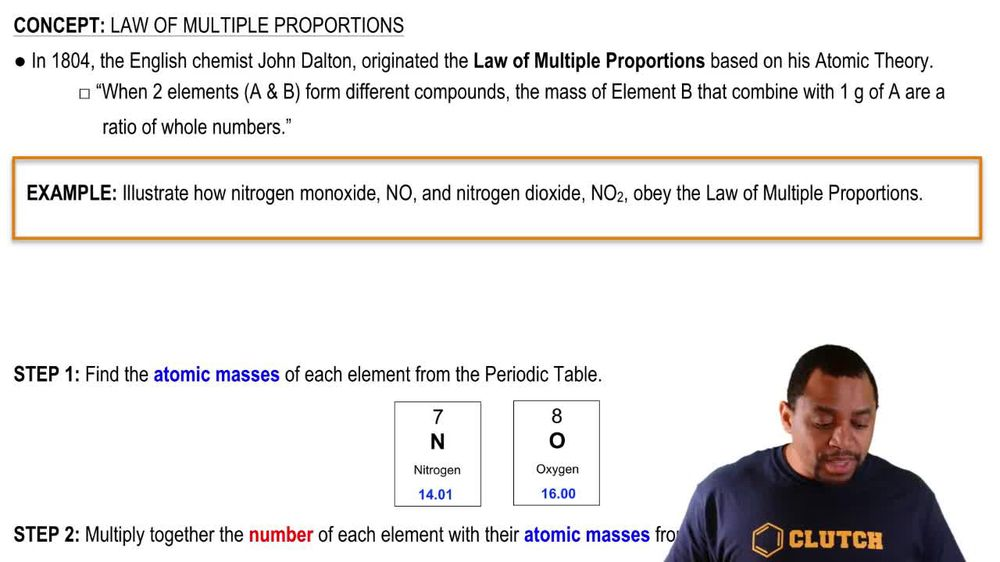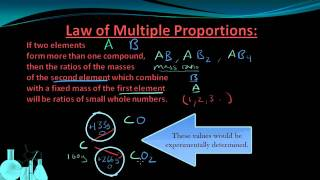Start typing, then use the up and down arrows to select an option from the list.
1. 2. Atoms & Elements2. Law of Multiple Proportions
Problem

# The ratio of the mass of a nitrogen atom to the mass of an atom of 12C is 7:6, and the ratio of the mass of nitrogen to oxygen in N2O is 7:4. Find the mass of 1 mol of oxygen atoms.

Relevant Solution3m
Play a video:
Hello everyone. Today's the question asks, Silicon di sulfide is a promising new material for the production of Catholics in solid state batteries. The mass ratio of silicon, sulfur and silicon di sulfide is 7 to 8 while the ratio of masses between the sulfur atom and the carbon 12 atom is 8 to 3. What is the mass of one mole of silicon atoms? So first we need to note the ratio between sulfur and carbon 12. The ratio between sulfur and carbon 12 estimated in the question Is 8.3. Or in other words we can say this is also going to be equal 16-6. We just multiply two to each compound. So we then find the ratio of silicon to sulfur. Ask We know that this should be seven 2 simply because we have seven silicon from the question stem And for sulfur we have 16 on the left bare. This ultimately means So thus three show of carbon 12 to silicon is now six 27. Now we must find the mass of one mole of silicon atoms and that is going to be equal to first we're going to take one atom, one Carbon 12 Atom. We're gonna place that over one sulfur atom. We're gonna multiply by avocados number which is 6.02 times 10 to the 23rd power and say that those atoms there is equal to one mole of sulfur. We are then multiply that buy avocados number but this time on the denominator. Never gonna say this is in terms of our carbon 12. So one mole of carbon 12 is equal to 6.2 times 10 to the 23rd atoms of carbon. We're then going to bring this equation down here. We're going to multiply that number By the multiple ratio by saying two moles of Sulfur equal one mole of Silicon. As we see in this equation in the question stem almost done here, are they going to multiply that ratio by the molar mass of carbon 12. So 12 g of carbon 12 equals one mole Of carbon 12. And lastly, we're going to multiply by our ratio of silicon to sulfur or carbon. So we have seven g of silicon to six g of carbon 12. And so our units are all going to cancel out, cancel those. We can cancel these, we can cancel these and essentially, you want to be left with grams of silicon over moles of silicon. So this is going to be equal to 28 g of silicon over one mole of silicon. As our final answer. I hope this helped handle next time